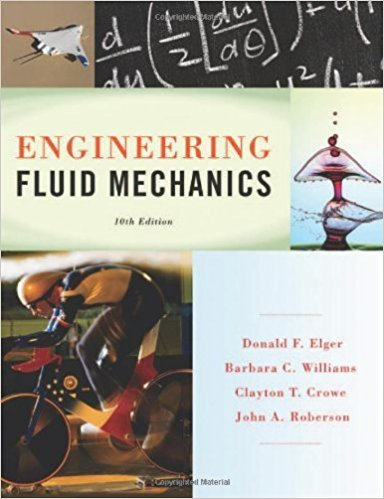×

# Which of the following could be considered a model Why (select all that apply). a. TheISBN: 9781118164297 288

## Solution for problem 16.1 Chapter 16

Engineering Fluid Mechanics | 10th Edition

• Textbook Solutions
• 2901 Step-by-step solutions solved by professors and subject experts
• Get 24/7 help from StudySoup virtual teaching assistantsEngineering Fluid Mechanics | 10th Edition

4 5 0 249 Reviews
23
5
Problem 16.1

Which of the following could be considered a model? Why? (select all that apply). a. The ideal-gas law b. A set of instructions for using a pitot-static tube to measure velocity c. An airplane built from a kit d. A computer program to predict the force on a pipe bend

Step-by-Step Solution:
Step 1 of 3

▯ ▯▯▯ ▯▯▯▯▯ ▯▯ ▯ ▯▯▯▯▯▯ ▯▯▯ ▯▯▯ ▯▯▯▯▯▯▯▯▯▯ ▯▯ ▯ ▯ ▯▯▯▯▯ ▯ ▯▯▯▯ ▯▯▯▯▯▯...

Step 2 of 3

Step 3 of 3

##### ISBN: 9781118164297

This full solution covers the following key subjects: . This expansive textbook survival guide covers 16 chapters, and 1172 solutions. The full step-by-step solution to problem: 16.1 from chapter: 16 was answered by , our top Physics solution expert on 01/25/18, 04:53PM. The answer to “Which of the following could be considered a model? Why? (select all that apply). a. The ideal-gas law b. A set of instructions for using a pitot-static tube to measure velocity c. An airplane built from a kit d. A computer program to predict the force on a pipe bend” is broken down into a number of easy to follow steps, and 50 words. Since the solution to 16.1 from 16 chapter was answered, more than 225 students have viewed the full step-by-step answer. This textbook survival guide was created for the textbook: Engineering Fluid Mechanics, edition: 10. Engineering Fluid Mechanics was written by and is associated to the ISBN: 9781118164297.

Unlock Textbook Solution

Which of the following could be considered a model Why (select all that apply). a. The

×